# Quantitative Imaging

The focus of this subproject is to significantly advance existing multi-modal PAT/OCT technology in order to extract quantitative information as well as biomechanical (elastographic) tissue parameters. Quantitative multi-modal PAT/OCT focuses on accurate detection of optical properties of the human epithelium and their changes for significantly improved understanding of cancerogenesis as well as improved early diagnosis of dysplasias to establish them as novel clinical diagnostic biomarkers for cancer diagnosis.

## Photoacoustic Tomography

In photoacoustic tomography, see for example the reference work , a sample is studied by illuminating it with (in our case usually near-infrared) laser light. This laser beam is then (according to the photoacoustic effect) partially absorbed in the medium which produces a locally varying temperature rise (depending on the strength of the absorption) leading to a pressure inequality inside the sample. The re-establishing of the pressure equilibrium then causes an ultrasonic wave to run through the sample which is recorded outside the object. From these pressure measurements around the sample, we want to recover the optical properties of the material.

One way to mathematically model this experiment is to use Maxwell’s equations for the propagation of the electric field $$E$$ of the laser beam and treat the sample as a dielectric medium described by the electric susceptibility $$\chi$$. This yields the Lippmann-Schwinger integral equation for the inverse temporal Fourier transform $$\check E$$ of the electric field $$E$$:

$$\check E(\omega,x) = \check E_0(\omega,x)+\left(\frac{\omega^2}{c^2}+\mathrm{grad}\,\mathrm{div}\right)\int_{\mathbb R^3}\frac{\mathrm e^{\mathrm i\frac\omega c|x-y|}}{4\pi|x-y|}\,\check\chi(\omega,y)\check E(\omega,y)\mathrm dy,$$

where $$E_0$$ denotes the electric field produced by the laser. Then, using the linear wave equation for the pressure wave $$P$$, we end up with the equation system

$$\partial_{tt}P(t,x) = \Delta_xP(t,x)$$,
$$\partial_tP(0,x) = 0$$,
$$P(0,x) = \Gamma(x)\int_{-\infty}^\infty\mathrm{Im}(\check\chi(\omega,x))|\check E(\omega,x)|^2\mathrm d\omega$$,

where $$\Gamma$$ denotes the Grüneisen parameter, that is the proportionality coefficient between absorbed energy and induced pressure density.

From measurements $$m(t,\xi)=P(t,\xi)$$ for all $$t>0$$ and all points $$\xi\in S$$ on some surface $$S\subset\mathbb R^3$$, the aim is to recover the unknown material parameters $$\chi$$ and $$\Gamma$$. It has been shown, however, that although we can (even analytically for simple surfaces $$S$$) reconstruct by inverting the wave equation the initial pressure distribution $$P(0,\cdot)$$, see for example , there is not enough data for a reconstruction of $$\chi$$ and $$\Gamma$$, see for example  or .

## Optical Coherence Tomography

This method is a special variant of an optical scattering experiment. For a thorough treatise, we refer to  and give only a summary of the working principle: A laser beam is sent from a certain direction onto the sample, where it is scattered and back-reflected. The light which is scattered back to the incoming direction is then measured interferometrically by superimposing it with a copy of the incident laser pulse. From the measurements of the Fourier transform of the superposition, one recovers the back-scattered electric field $$E(t,\xi)$$ for all $$t>0$$ on all points $$\xi\in D$$ of a plane $$D\subset\mathbb R^3$$ in front of the object, see for example  for a more detailed explanation of this mathematical model.

## Multimodal Photoacoustic and Optical Coherence Tomography

Combining Photoacoustic and Optical Coherence Tomography by using the same initial laser pulse and measuring the acoustic response and the back-scattered light at the same time, we are left with the problem of reconstructing the parameters $$\chi$$ and $$\Gamma$$ from the initial pressure $$P(0,\cdot)$$, acquired in a first inversion step and the knowledge $$E(t,\xi)$$, $$(t,\xi)\in(0,\infty)\times D$$, of the scattered field, see .

1. Guillaume Bal, Hybrid Inverse Problems and Internal Functionals, In: Inverse Problems and Applications: Inside Out II, Edited by G. Uhlmann, Volume 60, Mathematical Sciences Research Institute Publications, Cambridge University Press, 2013, pages 325-268, isbn: 978-1-107-03201-9.
2. Guillaume Bal, Kui Ren, Multi-source quantitative photoacoustic tomography in a diffusive regime, Inverse Problems 27.7, 075003 (2011).
3. Wolfgang Drexler and James G. Fujimoto, editors, Optical Coherence Tomography. Technology and Applications, Second edition, Springer International Publishing, Switzerland, 2015, isbn: 978-3-319-06418-5.
4. Peter Elbau, Leonidas Mindrinos, and Otmar Scherzer, Mathematical Methods of Optical Coherence Tomography, In: Handbook of Mathematical Methods in Imaging, Edited by Otmar Scherzer, Springer, 2015, pages 1169-1204, isbn: 978-1-4939-0789-2.
5. Peter Elbau, Leonidas Mindrinos, and Otmar Scherzer, Inverse Problems of Combined Photoacoustic and Optical Coherence Tomography, In: Mathematical Methods in the Applied Sciences 40.3 (2017), pages 505-522.
6. Lihong V. Wang, editor, Photoacoustic Imaging and Spectroscopy, CRC Press, 2009, isbn: 978-1-4200-5991-5.
7. Minghua Xu and Lihong V. Wang, Universal Back-Projection Algorithm for Photoacoustic Computed Tomography, In: Physical Review E 71.1, 016706 (2005).

## Research Team

#### From Subproject: Multi-Modal Imaging

Medical University Vienna Center for Medical Physics and Biomedical Engineering
General Hospital Vienna, 4L Währinger Gürtel 18-20 1090 Vienna, Austria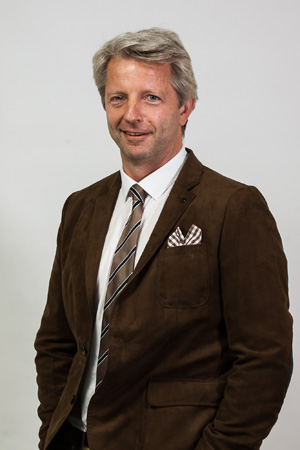Wolfgang DrexlerPrincipal Investigator Professor of Medical Physics Head of Center for Medical Physics and Biomedical Engineering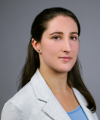Lisa KrainzPhD student

#### From Subproject: Quantitative Coupled Physics Imaging

University of Vienna Computational Science Center Oskar-Morgenstern-Platz 1 1090 Vienna, Austria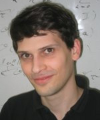Peter ElbauPrincipal Investigator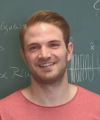Leopold VeselkaPhD student

#### From Subproject: Tomography with Uncertainties

University of Vienna Computational Science Center Oskar-Morgenstern-Platz 1 1090 Vienna, Austria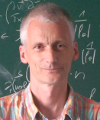Otmar ScherzerPrincipal Investigator Professor at the Faculty of Mathematics Computational Science Center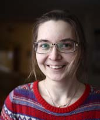Ekaterina SherinaPostdoctoral Researcher